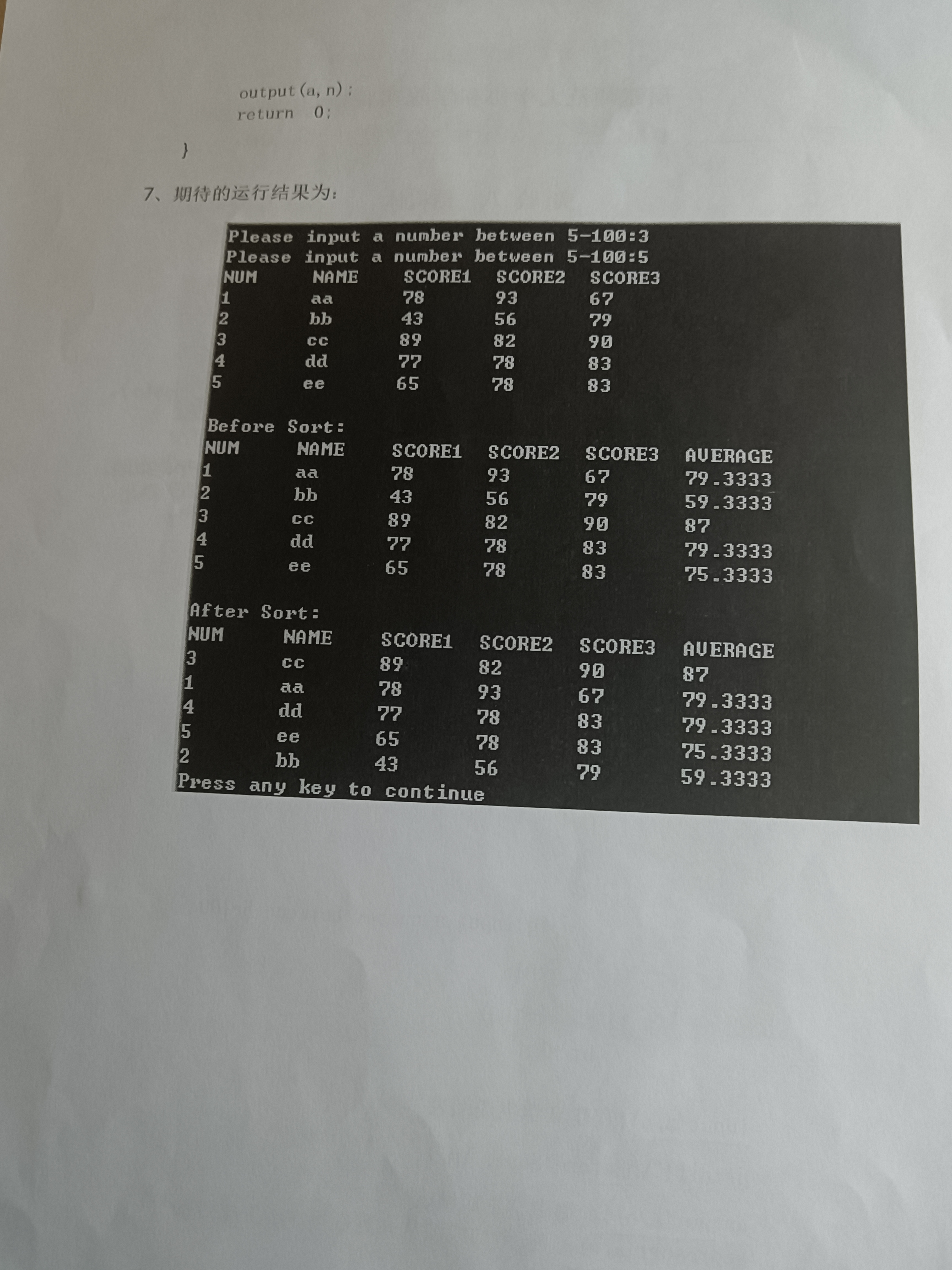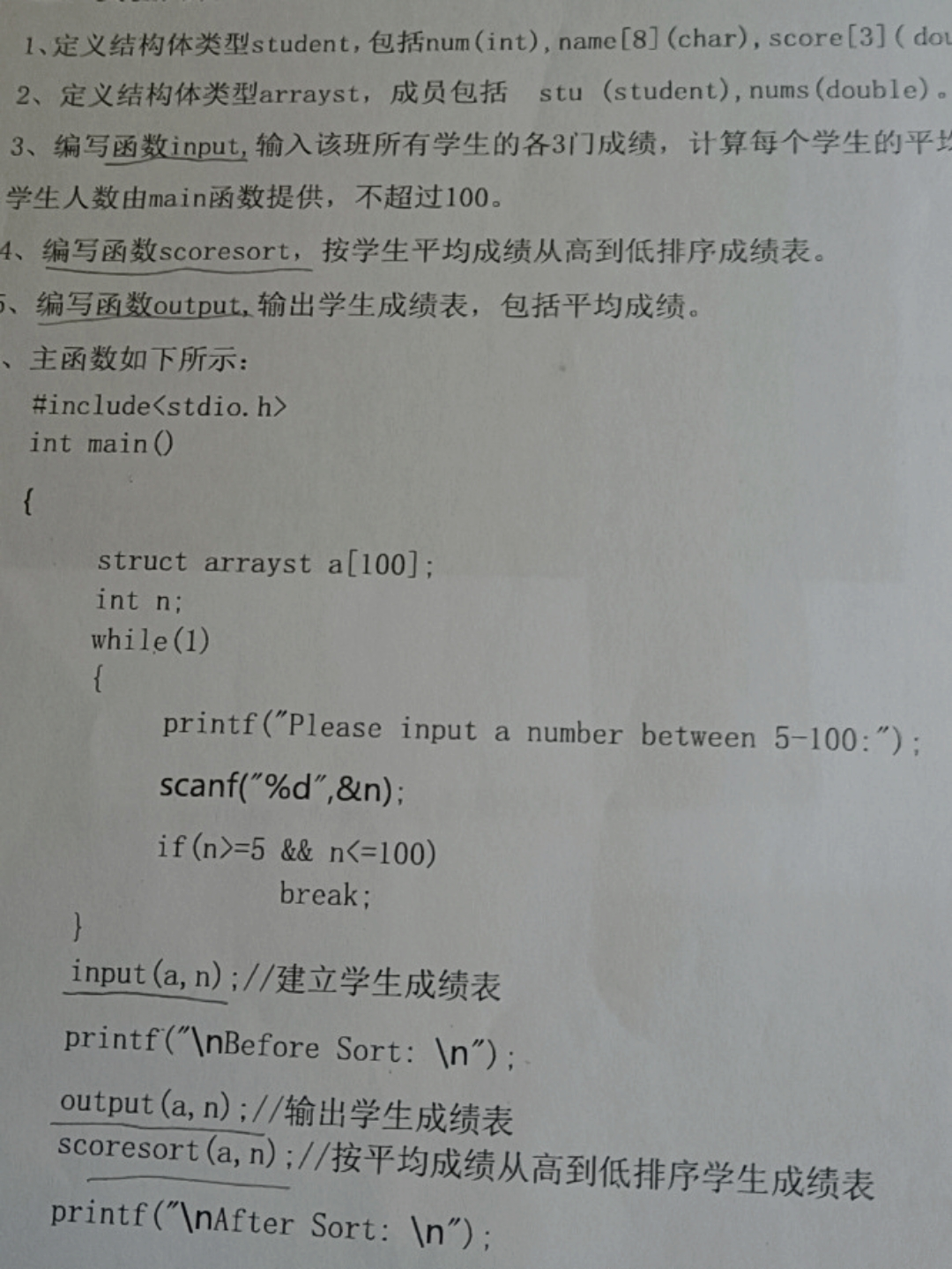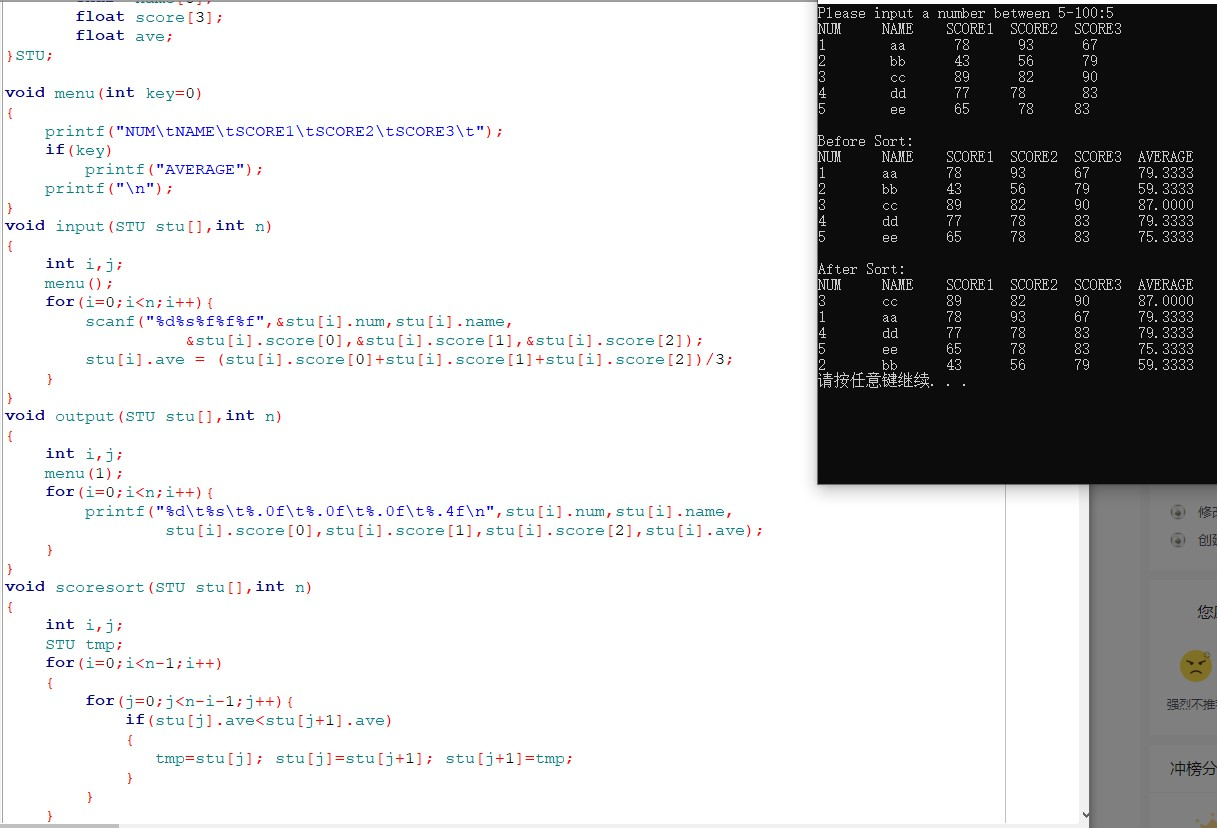2021-11-28 20:34

C语言利用结构体编程• 好问题 提建议
• 收藏

2条回答默认 最新

•qzjhjxj 2021-11-28 22:12
已采纳

供参考：

#include<stdio.h>
#define NUM   100
typedef struct student{
int   num;
char  name;
float score;
float ave;
}STU;

{
printf("NUM\tNAME\tSCORE1\tSCORE2\tSCORE3\t");
if(key)
printf("AVERAGE");
printf("\n");
}
void input(STU stu[],int n)
{
int i;
for(i=0;i<n;i++){
scanf("%d%s%f%f%f",&stu[i].num,stu[i].name,
&stu[i].score,&stu[i].score,&stu[i].score);
stu[i].ave = (stu[i].score+stu[i].score+stu[i].score)/3;
}
}
void output(STU stu[],int n)
{
int i;
for(i=0;i<n;i++){
printf("%d\t%s\t%.0f\t%.0f\t%.0f\t%.4f\n",stu[i].num,stu[i].name,
stu[i].score,stu[i].score,stu[i].score,stu[i].ave);
}
}
void scoresort(STU stu[],int n)
{
int i,j;
STU tmp;
for(i=0;i<n-1;i++)
{
for(j=0;j<n-i-1;j++){
if(stu[j].ave<stu[j+1].ave)
{
tmp=stu[j]; stu[j]=stu[j+1]; stu[j+1]=tmp;
}
}
}
}

int main()
{
STU stu[NUM];
int n;
while(1)
{
printf("Please input a number between 5-100:");
scanf("%d",&n);
if(n >= 5 && n <= 100) break;
}
input(stu,n);
printf("\nBefore Sort:\n");
output(stu,n);

scoresort(stu,n);
printf("\nAfter Sort:\n");
output(stu,n);

return 0;
}评论
解决 1 无用
打赏 举报
• 查看更多回答(1条)